|

# 这么简约实用的美式风,还是第一次见到!

今天与大家分享一套简约美式风案例，100㎡房子被简单划分为两室，整体上非常通透，呈现温暖的美式风格。在设计上简约而不简单。活泼中透出高贵。接下来就一起跟着编辑来欣赏业主的家。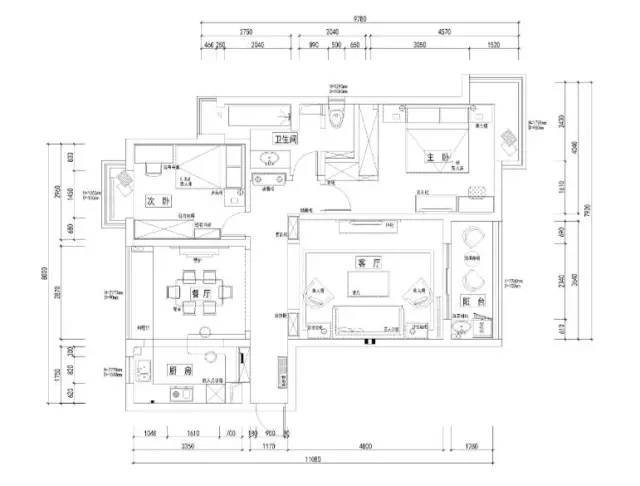客厅灰褐色背景下加入Tiffany蓝软装，充满高贵气质，这套案例半包设计花费11.3万元，主材、软装和家电共花费35万元。业主是对年轻小夫妻，在医疗机构工作，希望家中可以设计成温馨简约的美式风格，设计之前非常注重与设计师的沟通，业主详细介绍了自己的生活习惯，明确表示了自己对美式的理解，希望整体可以呈现浅咖色彩，也向设计师提供了一些参考图片，相当于业主列出框架，设计师将具体血肉填充进去。房间整体的设计非常注重线条感，可以看出客厅有罗马柱的设计，实际上那是一个造型柜，有一个面是门板，可以打开，里面可以挂衣物。做成这样的造型，是想增强空间的力度，又充满宫廷的华贵感。这种罗马柜的设计，可以用来进行区域划分，在业主家，就利用柜子将空间分隔为客厅、餐厅、门厅，也有更简洁的造型，对层高没有特别要求，基本的大户型都可以尝试这样的设计。

客厅基本上延续了HarborHouse的风格，家具采用了木色系，软装以大地色为主，感觉上更清新淡雅。客厅的颜色非常简洁统一，白色墙壁，亚麻色沙发，香槟色窗帘，浅棕色地板/电视柜和茶几，颜色由浅入深又相互碰撞，非常和谐有趣。以绿色抱枕和台灯作为点缀，为房间增添了美式田园风。客厅的金属色吊灯充满了宫廷式的贵气感，看出业主对细节非常关注。地板是欧洲进口的三层实木复合，品牌是金鹰爱格的三层实木复合地板，偏高端，价格在800~1000元。因为做了中央空调，如果再做地暖层高会相应减少十公分，所以再三考虑还是舍弃了地暖。

电视柜上的铜色复古闹钟，沙发边柜上的银色烟灰缸，精致的小物点缀，让房间更加耐人寻味。电视柜两侧的绿植和桌上的鲜花，又为家中增添了生机。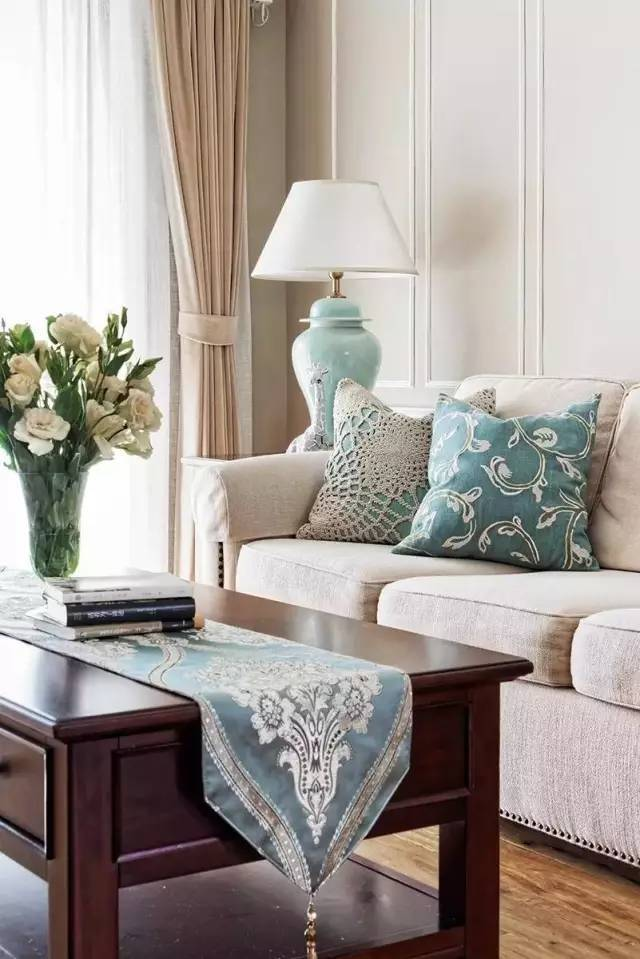茶几上的桌布与沙发上抱枕颜色相配，非常美观，桌布设计非常精致，小细节为房间增添了情趣和格调。

餐厅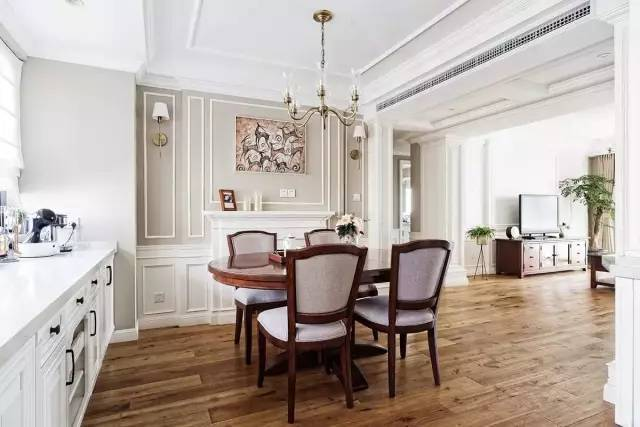因为客厅和餐厅有中央空调机子和风口，所以结合走道端景以及罗马柱做了假梁效果。餐厅与客厅基本位于一线，之间没有隔断，使房间看起来更大，整体通透。餐厅又与厨房连接，使得烹饪变得非常有趣方便,做饭的时候还可以与亲人互动，家中有宝宝的话，就可以边做饭边照看宝宝。做好的饭菜转身即可上桌，省时又方便。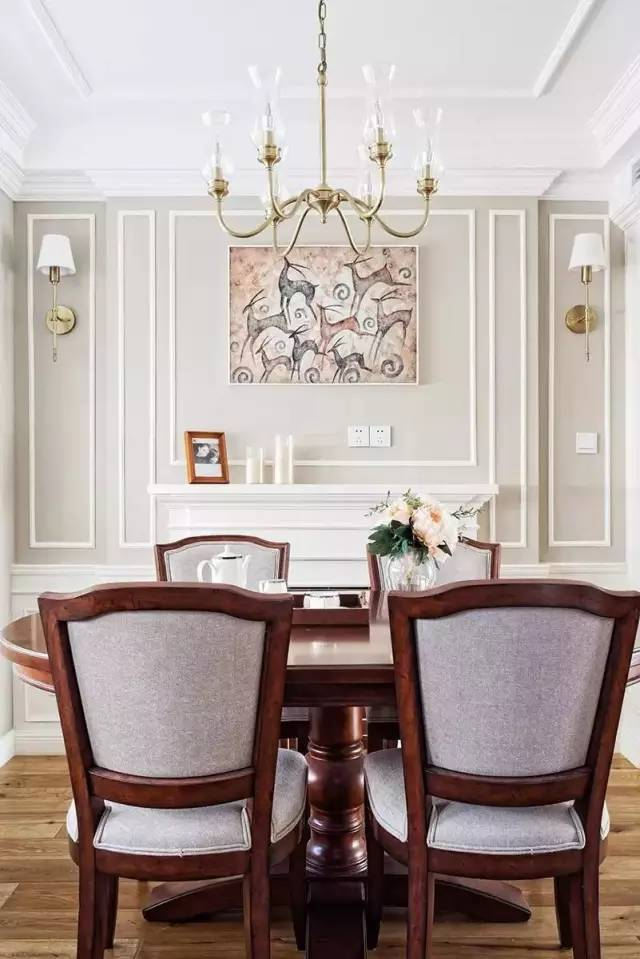餐厅桐黄色的吊灯，与客厅相似但细节又不同，有着宫廷风的贵族气息，又不繁复，颜色又与相近色的黄棕色餐桌和地板相互呼应，和谐美观。主灯和壁灯是HH的。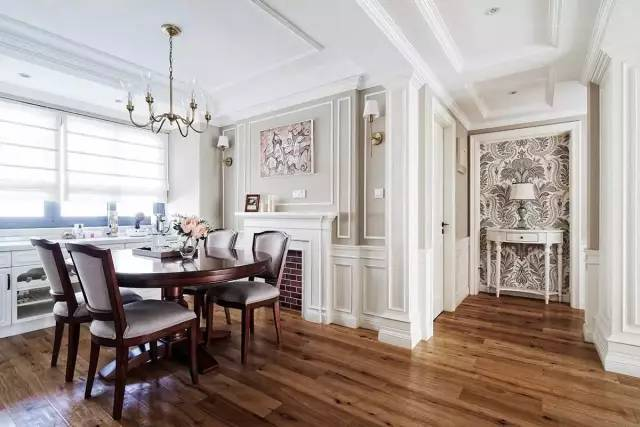餐桌另一边是美式壁炉设计，优雅美观。几何形线条的运用，充满大气华贵的artdeco风格，墙上的壁画是业主原有的收藏，说挂在房间里寓意比较好，而且颜色与餐桌上的香槟色鲜花又非常和谐搭配。两侧的两个桐色宫廷壁灯简约美观，夜晚又可以照亮局部，非常实用。餐桌有着中式实木家具的质感，设计上又突出了美式的设计感，椭圆形桌角非常光滑，线条流畅，中和了墙面和吊顶的竖线条，刚柔并济，突出美式风格的浪漫奔放。餐椅与餐桌完美搭配，增加了柔软坐垫，用餐时候会更加舒适。

餐厅靠近窗户，采光很好，即便不用餐，平时在这里会客、工作都是不错的选择。餐厅靠窗的部分增添了欧式储物柜，与厨房的橱柜是同一个品牌，颜色统一，而且白色会让家里更亮，可以用来收纳大件物品。业主选择了半透明白罗马帘，是家居装修中的一种新的型装饰品，有折叠式、扇形式、波浪式，可选择电动的，比较适合这种台上的窗户使用。拉上之后，既可以有效遮阳，又不会让家里显得过暗。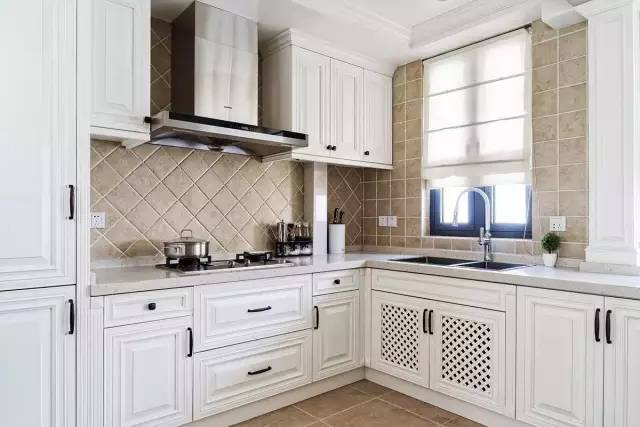把原来的北阳台归为室内，扩大了厨房空间，是西厨+餐边柜的概念，可以放很多的小电器和西点，非常实用。橱柜橱柜选择了比较擅长做美式的百益订制，墙砖的品牌是小蜜蜂。

业主喜欢空间通透的效果，做饭口味也比较清单，而且现在的油烟机也功率比较强大，所以决定使用开放式厨房。L型厨房很好地利用了拐角空间，橱柜的设计非常精致，增加了美式的线条感，又不会很复杂。镂空的设计很棒，既可以通风又美观。头顶的两个吊柜最大化利用了空间，也增加了储物空间。

厨房的地砖和墙上的壁砖统一呼应，浅棕色与房间整体颜色搭配，而且颜色非常饱满，中和了简单的白，让厨房更有层次感。

厨房改用壁砖，又简单地将餐厅与厨房分为了两个区域，进行了软隔断。厨房的窗帘也是半透明布艺卷帘，简单实用。操作台上摆一盆绿植，非常有生活情调。餐厅与厨房紧密相连，纯白操作台与之呼应，凝聚着整体格调。

玄关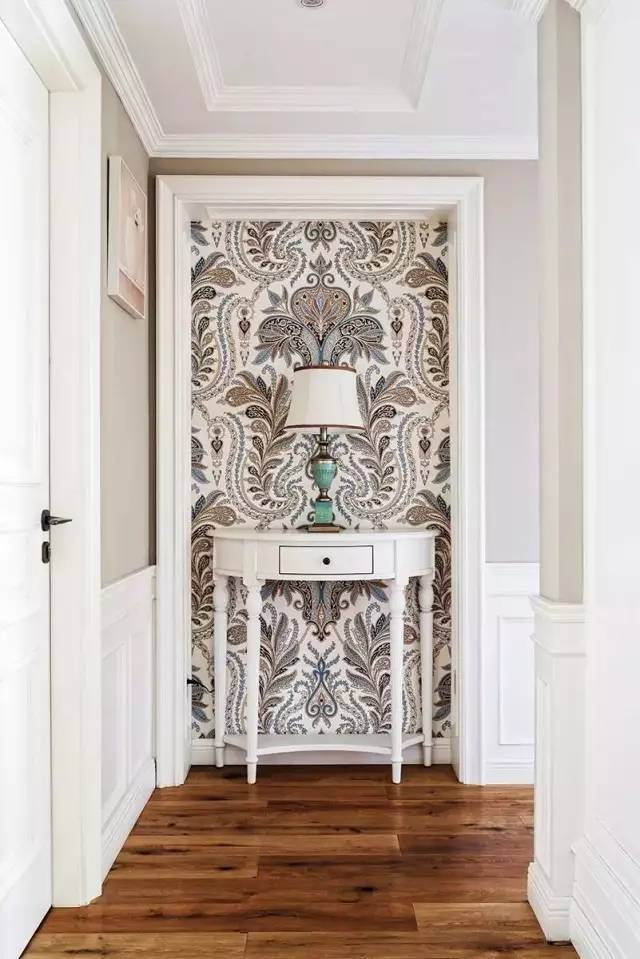玄关出的绚丽鸢尾壁纸吸引眼球，带来源源不断的浪漫气息，是业主在网上淘来的。一把欧式桌子和翠绿色宫廷台灯，成为视觉中心，非常有设计感。

卧室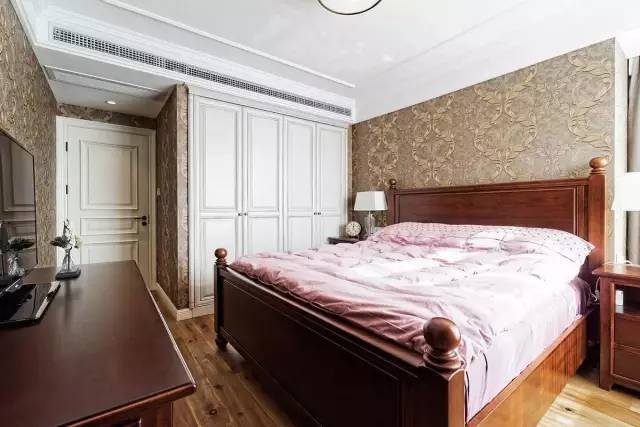主卧的设计非常特别，最吸引人的莫过于墙壁的装饰。全部以浅棕色壁纸包装，壁纸上的花纹有立体效果，很贵气，壁纸是业主网上淘宝。地板延伸了客厅了的地板铺装。

卧室的衣柜设计为嵌入式壁柜，有效节省了空间，白色的壁柜与卧室白色的们相呼应。暗棕色的美式乡村风床与面前的电脑桌相呼应，使得房间在色彩上多而不乱，而且充满层次感。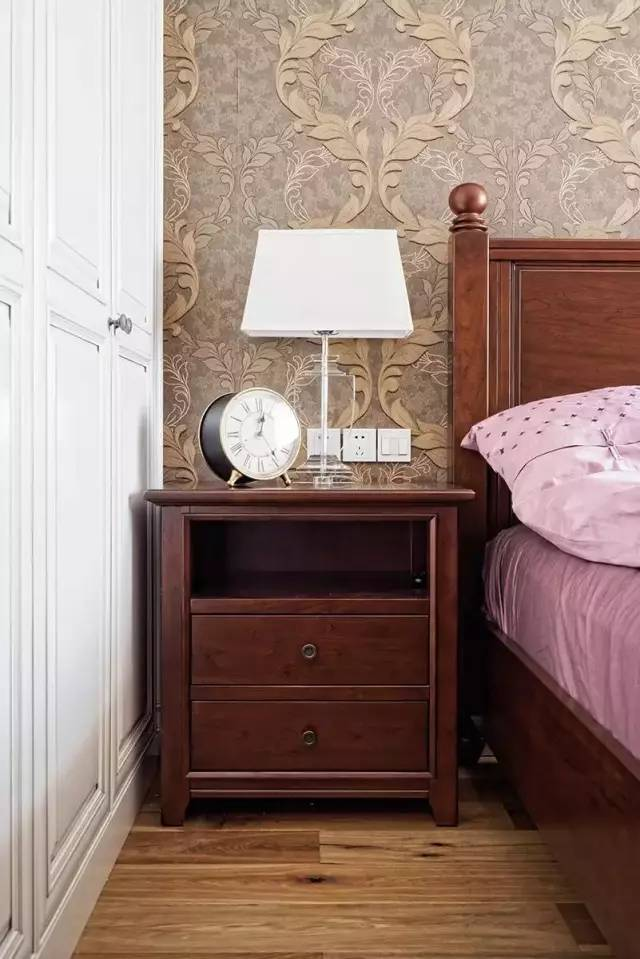床头柜的复古闹钟与客厅电视柜上的是同一款，完美融入了空间整体的复古感觉。灰色的窗帘弱化了存在感，让空间更和谐。

儿童房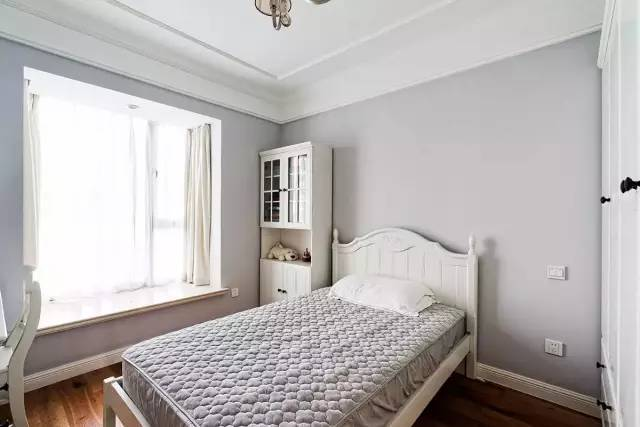儿童房很简单，浅灰色墙壁，让给房间变得很宁静，奶白色窗帘提亮了整个空间。原有的宽阔的飘窗，既可以用来储物，也可以坐在上面看书，天气晴好的日子，阳光洒进来，慵懒地坐在窗台前，是多么惬意的享受啊！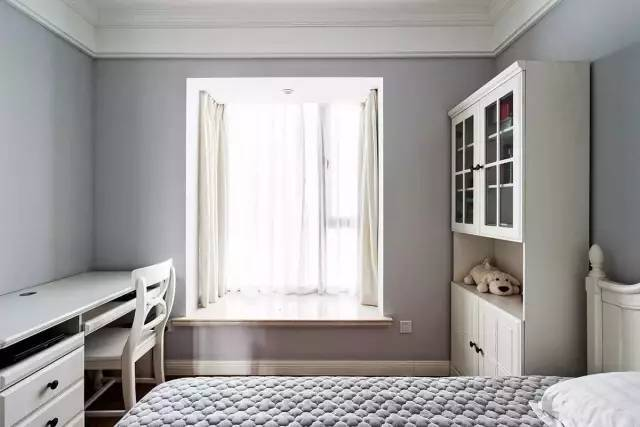儿童房内非常简洁，一个储物柜一张电脑桌，就可以满足需求。孩子活动的空间增大了，非常舒适。

卫生间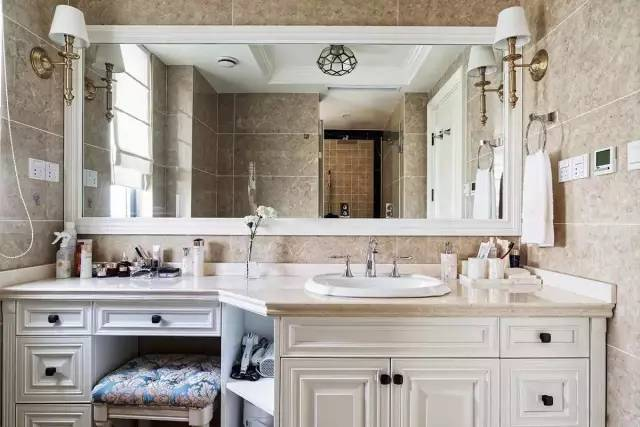一进入卫生间，就感觉进入了五星级酒店卫生间的感觉，巨大的梳妆镜非常抢眼，让洗手间的空间更加通透。左右两侧仍然是铜色宫廷风壁灯，洗浴间的顶灯是不规则几何形拼接的球形等，artdeco风格，美观简约大气。因为目前只有二人居住，所以业主希望卫生间能做的大一点，舒服一点，可以有浴缸和淋浴房及双台盆卫浴的一大特色便是将梳妆台与台盆柜结合，造型非常大气，有保证了空间的完整性节约了空间。柜子与厨房中的橱柜是同一种设计风格，连把手都非常精致。柜子的收纳做得也非常到位，连拐角处的空间都得到了有效利用。浅棕色壁砖的选择也与整体风格非常和谐搭配。

`声明：本文由入驻焦点开放平台的作者撰写，除焦点官方账号外，观点仅代表作者本人，不代表焦点立场错误信息举报电话： 400-099-0099，邮箱：jubao@vip.sohu.com，或点此进行意见反馈，或点此进行举报投诉。`A B C D E F G H J K L M N P Q R S T W X Y Z
A - B - C - D - E
• A
• 鞍山
• 安庆
• 安阳
• 安顺
• 安康
• 澳门
• B
• 北京
• 保定
• 包头
• 巴彦淖尔
• 本溪
• 蚌埠
• 亳州
• 滨州
• 北海
• 百色
• 巴中
• 毕节
• 保山
• 宝鸡
• 白银
• 巴州
• C
• 承德
• 沧州
• 长治
• 赤峰
• 朝阳
• 长春
• 常州
• 滁州
• 池州
• 长沙
• 常德
• 郴州
• 潮州
• 崇左
• 重庆
• 成都
• 楚雄
• 昌都
• 慈溪
• 常熟
• D
• 大同
• 大连
• 丹东
• 大庆
• 东营
• 德州
• 东莞
• 德阳
• 达州
• 大理
• 德宏
• 定西
• 儋州
• 东平
• E
• 鄂尔多斯
• 鄂州
• 恩施
F - G - H - I - J
• F
• 抚顺
• 阜新
• 阜阳
• 福州
• 抚州
• 佛山
• 防城港
• G
• 赣州
• 广州
• 桂林
• 贵港
• 广元
• 广安
• 贵阳
• 固原
• H
• 邯郸
• 衡水
• 呼和浩特
• 呼伦贝尔
• 葫芦岛
• 哈尔滨
• 黑河
• 淮安
• 杭州
• 湖州
• 合肥
• 淮南
• 淮北
• 黄山
• 菏泽
• 鹤壁
• 黄石
• 黄冈
• 衡阳
• 怀化
• 惠州
• 河源
• 贺州
• 河池
• 海口
• 红河
• 汉中
• 海东
• 怀来
• I
• J
• 晋中
• 锦州
• 吉林
• 鸡西
• 佳木斯
• 嘉兴
• 金华
• 景德镇
• 九江
• 吉安
• 济南
• 济宁
• 焦作
• 荆门
• 荆州
• 江门
• 揭阳
• 金昌
• 酒泉
• 嘉峪关
K - L - M - N - P
• K
• 开封
• 昆明
• 昆山
• L
• 廊坊
• 临汾
• 辽阳
• 连云港
• 丽水
• 六安
• 龙岩
• 莱芜
• 临沂
• 聊城
• 洛阳
• 漯河
• 娄底
• 柳州
• 来宾
• 泸州
• 乐山
• 六盘水
• 丽江
• 临沧
• 拉萨
• 林芝
• 兰州
• 陇南
• M
• 牡丹江
• 马鞍山
• 茂名
• 梅州
• 绵阳
• 眉山
• N
• 南京
• 南通
• 宁波
• 南平
• 宁德
• 南昌
• 南阳
• 南宁
• 内江
• 南充
• P
• 盘锦
• 莆田
• 平顶山
• 濮阳
• 攀枝花
• 普洱
• 平凉
Q - R - S - T - W
• Q
• 秦皇岛
• 齐齐哈尔
• 衢州
• 泉州
• 青岛
• 清远
• 钦州
• 黔南
• 曲靖
• 庆阳
• R
• 日照
• 日喀则
• S
• 石家庄
• 沈阳
• 双鸭山
• 绥化
• 上海
• 苏州
• 宿迁
• 绍兴
• 宿州
• 三明
• 上饶
• 三门峡
• 商丘
• 十堰
• 随州
• 邵阳
• 韶关
• 深圳
• 汕头
• 汕尾
• 三亚
• 三沙
• 遂宁
• 山南
• 商洛
• 石嘴山
• T
• 天津
• 唐山
• 太原
• 通辽
• 铁岭
• 泰州
• 台州
• 铜陵
• 泰安
• 铜仁
• 铜川
• 天水
• 天门
• W
• 乌海
• 乌兰察布
• 无锡
• 温州
• 芜湖
• 潍坊
• 威海
• 武汉
• 梧州
• 渭南
• 武威
• 吴忠
• 乌鲁木齐
X - Y - Z
• X
• 邢台
• 徐州
• 宣城
• 厦门
• 新乡
• 许昌
• 信阳
• 襄阳
• 孝感
• 咸宁
• 湘潭
• 湘西
• 西双版纳
• 西安
• 咸阳
• 西宁
• 仙桃
• 西昌
• Y
• 运城
• 营口
• 盐城
• 扬州
• 鹰潭
• 宜春
• 烟台
• 宜昌
• 岳阳
• 益阳
• 永州
• 阳江
• 云浮
• 玉林
• 宜宾
• 雅安
• 玉溪
• 延安
• 榆林
• 银川
• Z
• 张家口
• 镇江
• 舟山
• 漳州
• 淄博
• 枣庄
• 郑州
• 周口
• 驻马店
• 株洲
• 张家界
• 珠海
• 湛江
• 肇庆
• 中山
• 自贡
• 资阳
• 遵义
• 昭通
• 张掖
• 中卫

1室1厅1厨1卫1阳台

1
2
3
4
5

0
1
2

1

1

0
1
2
3报名成功，资料已提交审核A B C D E F G H J K L M N P Q R S T W X Y Z
A - B - C - D - E
• A
• 鞍山
• 安庆
• 安阳
• 安顺
• 安康
• 澳门
• B
• 北京
• 保定
• 包头
• 巴彦淖尔
• 本溪
• 蚌埠
• 亳州
• 滨州
• 北海
• 百色
• 巴中
• 毕节
• 保山
• 宝鸡
• 白银
• 巴州
• C
• 承德
• 沧州
• 长治
• 赤峰
• 朝阳
• 长春
• 常州
• 滁州
• 池州
• 长沙
• 常德
• 郴州
• 潮州
• 崇左
• 重庆
• 成都
• 楚雄
• 昌都
• 慈溪
• 常熟
• D
• 大同
• 大连
• 丹东
• 大庆
• 东营
• 德州
• 东莞
• 德阳
• 达州
• 大理
• 德宏
• 定西
• 儋州
• 东平
• E
• 鄂尔多斯
• 鄂州
• 恩施
F - G - H - I - J
• F
• 抚顺
• 阜新
• 阜阳
• 福州
• 抚州
• 佛山
• 防城港
• G
• 赣州
• 广州
• 桂林
• 贵港
• 广元
• 广安
• 贵阳
• 固原
• H
• 邯郸
• 衡水
• 呼和浩特
• 呼伦贝尔
• 葫芦岛
• 哈尔滨
• 黑河
• 淮安
• 杭州
• 湖州
• 合肥
• 淮南
• 淮北
• 黄山
• 菏泽
• 鹤壁
• 黄石
• 黄冈
• 衡阳
• 怀化
• 惠州
• 河源
• 贺州
• 河池
• 海口
• 红河
• 汉中
• 海东
• 怀来
• I
• J
• 晋中
• 锦州
• 吉林
• 鸡西
• 佳木斯
• 嘉兴
• 金华
• 景德镇
• 九江
• 吉安
• 济南
• 济宁
• 焦作
• 荆门
• 荆州
• 江门
• 揭阳
• 金昌
• 酒泉
• 嘉峪关
K - L - M - N - P
• K
• 开封
• 昆明
• 昆山
• L
• 廊坊
• 临汾
• 辽阳
• 连云港
• 丽水
• 六安
• 龙岩
• 莱芜
• 临沂
• 聊城
• 洛阳
• 漯河
• 娄底
• 柳州
• 来宾
• 泸州
• 乐山
• 六盘水
• 丽江
• 临沧
• 拉萨
• 林芝
• 兰州
• 陇南
• M
• 牡丹江
• 马鞍山
• 茂名
• 梅州
• 绵阳
• 眉山
• N
• 南京
• 南通
• 宁波
• 南平
• 宁德
• 南昌
• 南阳
• 南宁
• 内江
• 南充
• P
• 盘锦
• 莆田
• 平顶山
• 濮阳
• 攀枝花
• 普洱
• 平凉
Q - R - S - T - W
• Q
• 秦皇岛
• 齐齐哈尔
• 衢州
• 泉州
• 青岛
• 清远
• 钦州
• 黔南
• 曲靖
• 庆阳
• R
• 日照
• 日喀则
• S
• 石家庄
• 沈阳
• 双鸭山
• 绥化
• 上海
• 苏州
• 宿迁
• 绍兴
• 宿州
• 三明
• 上饶
• 三门峡
• 商丘
• 十堰
• 随州
• 邵阳
• 韶关
• 深圳
• 汕头
• 汕尾
• 三亚
• 三沙
• 遂宁
• 山南
• 商洛
• 石嘴山
• T
• 天津
• 唐山
• 太原
• 通辽
• 铁岭
• 泰州
• 台州
• 铜陵
• 泰安
• 铜仁
• 铜川
• 天水
• 天门
• W
• 乌海
• 乌兰察布
• 无锡
• 温州
• 芜湖
• 潍坊
• 威海
• 武汉
• 梧州
• 渭南
• 武威
• 吴忠
• 乌鲁木齐
X - Y - Z
• X
• 邢台
• 徐州
• 宣城
• 厦门
• 新乡
• 许昌
• 信阳
• 襄阳
• 孝感
• 咸宁
• 湘潭
• 湘西
• 西双版纳
• 西安
• 咸阳
• 西宁
• 仙桃
• 西昌
• Y
• 运城
• 营口
• 盐城
• 扬州
• 鹰潭
• 宜春
• 烟台
• 宜昌
• 岳阳
• 益阳
• 永州
• 阳江
• 云浮
• 玉林
• 宜宾
• 雅安
• 玉溪
• 延安
• 榆林
• 银川
• Z
• 张家口
• 镇江
• 舟山
• 漳州
• 淄博
• 枣庄
• 郑州
• 周口
• 驻马店
• 株洲
• 张家界
• 珠海
• 湛江
• 肇庆
• 中山
• 自贡
• 资阳
• 遵义
• 昭通
• 张掖
• 中卫• 手机• 分享
• 设计
免费设计
• 计算器
装修计算器
• 入驻
合作入驻
• 联系
联系我们
• 置顶
返回顶部